SLVSAP3D December   2010  – February 2016

PRODUCTION DATA.

1. Features
2. Applications
3. Description
4. Revision History
5. Pin Configuration and Functions
6. Specifications
7. Detailed Description
8. Application and Implementation
1. 8.1 Application Information
2. 8.2 Typical Application
1. 8.2.1 Design Requirements
2. 8.2.2 Detailed Design Procedure
3. 8.2.3 Application Curves
9. Power Supply Recommendations
10. 10Layout
11. 11Device and Documentation Support
1. 11.1 Device Support
2. 11.2 Documentation Support
3. 11.3 Community Resource
5. 11.5 Electrostatic Discharge Caution
6. 11.6 Glossary
12. 12Mechanical, Packaging, and Orderable Information

• DGQ|10
• DRC|10
• DGQ|10
• DRC|10

## 8 Application and Implementation

NOTE

Information in the following applications sections is not part of the TI component specification, and TI does not warrant its accuracy or completeness. TI’s customers are responsible for determining suitability of components for their purposes. Customers should validate and test their design implementation to confirm system functionality.

### 8.1 Application Information

The TPS57140-Q1 DC-DC converter is designed to provide up to a 1.5-A output from an input voltage source of 3.5 V to 42 V. The high-side MOSFET is incorporated inside the TPS57140-Q1 package along with the gate drive circuitry. The low drain-to-source on-resistance of the MOSFET allows the TPS57140-Q1 device to achieve high efficiencies and helps keep the junction temperature low at high output currents. The compensation components are external to the integrated circuit (IC), and an external divider allows for an adjustable output voltage. Additionally, the TPS57140-Q1 device provides adjustable slow start and undervoltage-lockout inputs.

### 8.2 Typical Application

This example details the design of a high frequency switching regulator design using ceramic output capacitors. A few parameters must be known to start the design process. These parameters are typically determined at the system level.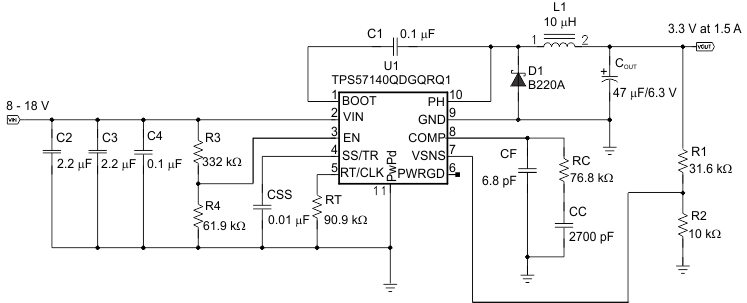Figure 51. High-Frequency, 3.3-V Output Power-Supply Design With Adjusted UVLO

#### 8.2.1 Design Requirements

For this example, start with the known parameters listed in Table 1.

### Table 1. Design Parameters

DESIGN PARAMETER EXAMPLE VALUE
Output voltage 3.3 V
Transient response 0 to 1.5-A load step ΔVOUT = 4%
Maximum output current 1.5 A
Input voltage 12 V (nominal), 8 V to 18 V
Output voltage ripple < 33 mVpp
Start input voltage (rising VIN) 7.25 V
Stop input voltage (falling VIN) 6.25 V

#### 8.2.2.1 Selecting the Switching Frequency

The first step is to decide on a switching frequency for the regulator. Typically, the user wants to choose the highest switching frequency possible, because this produces the smallest solution size. The high switching frequency allows for lower valued inductors and smaller output capacitors compared to a power supply that switches at a lower frequency. The switching frequency that can be selected is limited by the minimum on-time of the internal power switch, the input voltage and the output voltage and the frequency shift limitation.

Equation 8 and Equation 9 must be used to find the maximum switching frequency for the regulator, choose the lower value of the two equations. Switching frequencies higher than these values result in pulse skipping or the lack of overcurrent protection during a short circuit.

The typical minimum on time (tonmin) is 130 ns for the TPS57140-Q1 device. For this example, the output voltage is 3.3 V and the maximum input voltage is 18 V, which allows for a maximum switch frequency up to 1600 kHz when including the inductor resistance, on resistance and diode voltage in Equation 8. To ensure overcurrent runaway is not a concern during short circuits in your design use Equation 9 or the solid curve in Figure 33 to determine the maximum switching frequency. With a maximum input voltage of 20 V, for some margin above 18 V, assuming a diode voltage of 0.5 V, inductor resistance of 100 mΩ, switch resistance of 200 mΩ, a current limit value of 2.7 A, the maximum switching frequency is approximately 2500 kHz.

Choosing the lower of the two values and adding some margin a switching frequency of 1200 kHz is used. To determine the timing resistance for a given switching frequency, use Equation 7 or the curve in Figure 31.

The switching frequency is set by resistor Rt shown in Figure 51.

#### 8.2.2.2 Output Inductor Selection (LO)

To calculate the minimum value of the output inductor, use Equation 28.

KIND is a coefficient that represents the amount of inductor ripple current relative to the maximum output current.

The inductor ripple current is filtered by the output capacitor. Therefore, choosing high inductor ripple currents impacts the selection of the output capacitor, because the output capacitor must have a ripple current rating equal to or greater than the inductor ripple current. In general, the inductor ripple value is at the discretion of the designer; however, the following guidelines may be used.

For designs using low ESR output capacitors such as ceramics, a value as high as KIND = 0.3 may be used. When using higher ESR output capacitors, KIND = 0.2 yields better results. Because the inductor ripple current is part of the PWM control system, the inductor ripple current should always be greater than 100 mA for dependable operation. In a wide input voltage regulator, it is best to choose an inductor ripple current on the larger side. This allows the inductor to still have a measurable ripple current with the input voltage at its minimum.

For this design example, use KIND = 0.2 and the minimum inductor value is calculated to be 7.6 μH. For this design, a nearest standard value was chosen: 10 μH. For the output filter inductor, it is important that the RMS current and saturation current ratings not be exceeded. The RMS and peak inductor current can be found from Equation 30 and Equation 31.

For this design, the RMS inductor current is 1.506 A and the peak inductor current is 1.62 A. The chosen inductor is a MSS6132-103. It has a saturation current rating of 1.64 A and an RMS current rating of 1.9 A.

As the equation set demonstrates, lower ripple currents reduce the output voltage ripple of the regulator but require a larger value of inductance. Selecting higher ripple currents increases the output voltage ripple of the regulator but allows for a lower inductance value.

The current flowing through the inductor is the inductor ripple current plus the output current. During power up, faults or transient load conditions, the inductor current can increase above the calculated peak inductor current level calculated above. In transient conditions, the inductor current can increase up to the switch current limit of the device. For this reason, the most conservative approach is to specify an inductor with a saturation current rating equal to or greater than the switch current limit rather than the peak inductor current.

Equation 28.Equation 29.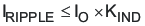Equation 30.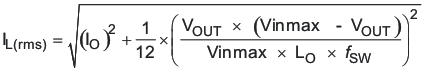Equation 31.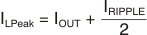#### 8.2.2.3 Output Capacitor

There are three primary considerations for selecting the value of the output capacitor. The output capacitor determines the modulator pole, the output voltage ripple, and how the regulators responds to a large change in load current. The output capacitance needs to be selected based on the more stringent of these three criteria.

The desired response to a large change in the load current is the first criteria. The output capacitor needs to supply the load with current when the regulator cannot. This situation would occur if there are desired hold-up times for the regulator where the output capacitor must hold the output voltage above a certain level for a specified amount of time after the input power is removed. The regulator also temporarily is not able to supply sufficient output current if there is a large fast increase in the current needs of the load such as transitioning from no load to a full load. The regulator usually needs two or more clock cycles for the control loop to see the change in load current and output voltage and adjust the duty cycle to react to the change. The output capacitor must be sized to supply the extra current to the load until the control loop responds to the load change. The output capacitance must be large enough to supply the difference in current for two clock cycles while only allowing a tolerable amount of droop in the output voltage. Equation 32 shows the minimum output capacitance necessary to accomplish this.

Where ΔIOUT is the change in output current, ƒsw is the regulators switching frequency and ΔVOUT is the allowable change in the output voltage. For this example, the transient load response is specified as a 4% change in VOUT for a load step from 0 A (no load) to 1.5 A (full load). For this example, ΔIOUT = 1.5 – 0 = 1.5 A and ΔVOUT = 0.04 × 3.3 = 0.132 V. Using these numbers gives a minimum capacitance of 18.9 μF. This value does not take the ESR of the output capacitor into account in the output voltage change. For ceramic capacitors, the ESR is usually small enough to ignore in this calculation. Aluminum electrolytic and tantalum capacitors have higher ESR that should be taken into account.

The catch diode of the regulator cannot sink current, so any stored energy in the inductor produces an output voltage overshoot when the load current rapidly decreases (see Figure 52). The output capacitor must be sized to absorb energy stored in the inductor when transitioning from a high load current to a lower load current. The excess energy that is stored in the output capacitor increases the voltage on the capacitor. The capacitor must be sized to maintain the desired output voltage during these transient periods. Equation 33 is used to calculate the minimum capacitance to keep the output voltage overshoot to a desired value. Where L is the value of the inductor, IOH is the output current under heavy load, IOL is the output under light load, VF is the final peak output voltage, and Vi is the initial capacitor voltage. For this example, the worst-case load step is from 1.5 A to 0 A. The output voltage increases during this load transition, and the stated maximum output voltage in the specification is 4% of the nominal output voltage. This makes VF = 1.04 × 3.3 = 3.432. Vi is the initial capacitor voltage, which is the nominal output voltage of 3.3 V. Using these numbers in Equation 33 yields a minimum capacitance of 25.3 μF.

Equation 34 calculates the minimum output capacitance needed to meet the output voltage ripple specification. Where ƒsw is the switching frequency, Voripple is the maximum allowable output voltage ripple, and Iripple is the inductor ripple current. Equation 35 yields 0.7 μF.

Equation 35 calculates the maximum ESR an output capacitor can have to meet the output voltage ripple specification. Equation 35 indicates the ESR should be less than 147 mΩ.

The most stringent criteria for the output capacitor is 25.3 μF of capacitance to keep the output voltage in regulation during an unload transient.

Additional capacitance de-ratings for aging, temperature, and dc bias should be factored in, which increases this minimum value. For this example, a 47-μF 6.3-V X7R ceramic capacitor with 5-mΩ ESR is used.

Capacitors generally have limits to the amount of ripple current they can handle without failing or producing excess heat. An output capacitor that can support the inductor ripple current must be specified. Some capacitor data sheets specify the root mean square (RMS) value of the maximum ripple current. Equation 36 can be used to calculate the RMS ripple current the output capacitor needs to support. For this application, Equation 36 yields 64.8 mA.

Equation 32.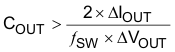Equation 33.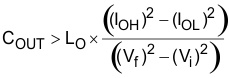Equation 34.Equation 35.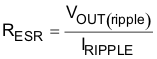Equation 36.#### 8.2.2.4 Catch Diode

The TPS57140-Q1 device requires an external catch diode between the PH pin and GND. The selected diode must have a reverse voltage rating equal to or greater than VIN(max). The peak current rating of the diode must be greater than the maximum inductor current. The diode should also have a low forward voltage. Schottky diodes are typically a good choice for the catch diode due to their low forward voltage. The lower the forward voltage of the diode, the higher the efficiency of the regulator.

Typically, the higher the voltage and current ratings the diode has, the higher the forward voltage. Because the design example has an input voltage up to 18 V, a diode with a minimum of 20-V reverse voltage is selected.

For the example design, the B220A Schottky diode is selected for its lower forward voltage, and it comes in a larger package size, which has good thermal characteristics over small devices. The typical forward voltage of the B220A is 0.5 V.

The diode must also be selected with an appropriate power rating. The diode conducts the output current during the off-time of the internal power switch. The off-time of the internal switch is a function of the maximum input voltage, the output voltage, and the switching frequency. The output current during the off-time is multiplied by the forward voltage of the diode which equals the conduction losses of the diode. At higher switch frequencies, the ac losses of the diode need to be taken into account. The ac losses of the diode are due to the charging and discharging of the junction capacitance and reverse recovery. Equation 37 is used to calculate the total power dissipation, conduction losses plus ac losses, of the diode.

The B220A has a junction capacitance of 120 pF. Using Equation 37, the selected diode dissipates 0.632 W. This power dissipation, depending on mounting techniques, should produce a 16°C temperature rise in the diode when the input voltage is 18 V and the load current is 1.5 A.

If the power supply spends a significant amount of time at light load currents or in sleep mode consider using a diode which has a low leakage current and slightly higher forward voltage drop.

Equation 37.#### 8.2.2.5 Input Capacitor

The TPS57140-Q1 device requires a high-quality ceramic, type X5R or X7R, input decoupling capacitor of at least 3-μF effective capacitance and, in some applications, a bulk capacitance. The effective capacitance includes any dc bias effects. The voltage rating of the input capacitor must be greater than the maximum input voltage. The capacitor must also have a ripple current rating greater than the maximum input current ripple of the TPS57140-Q1. The input ripple current can be calculated using Equation 38.

The value of a ceramic capacitor varies significantly over temperature and the amount of dc bias applied to the capacitor. The capacitance variations due to temperature can be minimized by selecting a dielectric material that is stable over temperature. X5R and X7R ceramic dielectrics are usually selected for power regulator capacitors because they have a high capacitance to volume ratio and are fairly stable over temperature. The output capacitor must also be selected with the dc bias taken into account. The capacitance value of a capacitor decreases as the dc bias across a capacitor increases.

For this example design, a ceramic capacitor with at least a 20-V voltage rating is required to support the maximum input voltage. Common standard ceramic capacitor voltage ratings include 4 V, 6.3 V, 10 V, 16 V, 25 V, 50 V or 100 V, so a 25-V capacitor should be selected. For this example, two 2.2-μF 25-V capacitors in parallel have been selected. Table 2 shows a selection of high voltage capacitors. The input capacitance value determines the input ripple voltage of the regulator. The input voltage ripple can be calculated using Equation 39. Using the design example values, Ioutmax = 1.5 A, CIN = 4.4 μF, ƒsw = 1200 kHz, yields an input voltage ripple of 71 mV and an RMS input ripple current of 0.701 A.

Equation 38.Equation 39.### Table 2. Capacitor Types

VENDOR VALUE (μF) EIA SIZE VOLTAGE DIELECTRIC COMMENTS
Murata 1 to 2.2 1210 100 V X7R GRM32 series
1 to 4.7 50 V
1 1206 100 V GRM31 series
1 to 2.2 50 V
Vishay 1 10 1.8 2220 50 V VJ X7R series
1 to 1.2 100 V
1 to 3.9 2225 50 V
1 to 1.8 100 V
TDK 1 to 2.2 1812 100 V C series C4532
1.5 to 6.8 50 V
1 to 2.2 1210 100 V C series C3225
1 to 3.3 50 V
AVX 1 to 4.7 1210 50 V X7R dielectric series
1 100 V
1 to 4.7 1812 50 V
1 to 2.2 100 V

#### 8.2.2.6 Slow-Start Capacitor

The slow-start capacitor determines the minimum amount of time required for the output voltage to reach its nominal programmed value during power up. This is useful if a load requires a controlled voltage slew rate. This is also used if the output capacitance is large and would require large amounts of current to quickly charge the capacitor to the output voltage level. The large currents necessary to charge the capacitor may make the TPS57140-Q1 reach the current limit or excessive current draw from the input power supply may cause the input voltage rail to sag. Limiting the output voltage slew rate solves both of these problems.

The slow start time must be long enough to allow the regulator to charge the output capacitor up to the output voltage without drawing excessive current. Equation 40 can be used to find the minimum slow start time, TSS, necessary to charge the output capacitor, COUT, from 10% to 90% of the output voltage, VOUT, with an average slow start current of ISSAVG. In the example, to charge the 47-μF output capacitor up to 3.3 V while only allowing the average input current to be 0.125 A requires a 1-ms slow start time.

Once the slow start time is known, the slow-start capacitor value can be calculated using Equation 6. For the example circuit, the slow start time is not too critical, because the output capacitor value is 47 μF which does not require much current to charge to 3.3 V. The example circuit has the slow start time set to an arbitrary value of 1ms which requires a 3.3-nF capacitor.

Equation 40.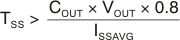#### 8.2.2.7 Bootstrap Capacitor Selection

A 0.1-μF ceramic capacitor must be connected between the BOOT and PH pins for proper operation. It is recommended to use a ceramic capacitor with X5R or better grade dielectric. The capacitor should have a 10-V or higher voltage rating.

#### 8.2.2.8 Undervoltage Lockout (UVLO) Set Point

The UVLO can be adjusted using an external voltage divider on the EN pin of the TPS57140-Q1. The UVLO has two thresholds, one for power up when the input voltage is rising and one for power down or brown outs when the input voltage is falling. For the example design, the supply should turn on and start switching once the input voltage increases above 7.25 V (enabled). After the regulator starts switching, it should continue to do so until the input voltage falls below 6.25 V (UVLO stop).

The programmable UVLO and enable voltages are set using a resistor divider between Vin and ground to the EN pin. Equation 2 through Equation 3 can be used to calculate the resistance values necessary. For the example application, a 332 kΩ between Vin and EN and a 61.9 kΩ between EN and ground are required to produce the 7.25 V and 6.25 V start and stop voltages.

#### 8.2.2.9 Output Voltage and Feedback Resistors Selection

For the example design, 10 kΩ was selected for R2. Using Equation 1, R1 is calculated as 31.25 kΩ. The nearest standard 1% resistor is 31.6 kΩ. Due to current leakage of the VSENSE pin, the current flowing through the feedback network should be greater than 1 μA to maintain the output voltage accuracy. This requirement makes the maximum value of R2 equal to 800 kΩ. Choosing higher resistor values decreases quiescent current and improves efficiency at low output currents but may introduce noise immunity problems.

#### 8.2.2.10 Compensation

There are several industry techniques used to compensate DC/DC regulators. The method presented here yields high phase margins. For most conditions, the regulator will have a phase margin between 60 and 90 degrees. The method presented here ignores the effects of the slope compensation that is internal to the TPS57140-Q1. Since the slope compensation is ignored, the actual crossover frequency is usually lower than the crossover frequency used in the calculations.

Use SwitcherPro software for a more accurate design.

The uncompensated regulator will have a dominant pole, typically located between 300 Hz and 3 kHz, due to the output capacitor and load resistance and a pole due to the error amplifier. One zero exists due to the output capacitor and the ESR. The zero frequency is higher than either of the two poles.

If left uncompensated, the double pole created by the error amplifier and the modulator would lead to an unstable regulator. To stabilize the regulator, one pole must be canceled out. One design approach is to locate a compensating zero at the modulator pole. Then select a crossover frequency that is higher than the modulator pole. The gain of the error amplifier can be calculated to achieve the desired crossover frequency. The capacitor used to create the compensation zero along with the output impedance of the error amplifier form a low frequency pole to provide a minus one slope through the crossover frequency. Then a compensating pole is added to cancel the zero due to the output capacitors ESR. If the ESR zero resides at a frequency higher than the switching frequency then it can be ignored.

To compensate the TPS57140-Q1 using this method, first calculate the modulator pole and zero using the following equations:

Equation 41.where

• IOUT(max) is the maximum output current
• COUT is the output capacitance
• VOUT is the nominal output voltage
Equation 42.For the example design, the modulator pole is located at 1.5 kHz and the ESR zero is located at 338 kHz.

Next, the designer selects a crossover frequency which will determine the bandwidth of the control loop. The crossover frequency must be located at a frequency at least five times higher than the modulator pole. The crossover frequency must also be selected so that the available gain of the error amplifier at the crossover frequency is high enough to allow for proper compensation.

Equation 47 is used to calculate the maximum crossover frequency when the ESR zero is located at a frequency that is higher than the desired crossover frequency. This will usually be the case for ceramic or low ESR tantalum capacitors. Aluminum Electrolytic and Tantalum capacitors will typically produce a modulator zero at a low frequency due to their high ESR.

The example application is using a low ESR ceramic capacitor with 10 mΩ of ESR making the zero at 338 kHz.

This value is much higher than typical crossover frequencies so the maximum crossover frequency is calculated using both Equation 43 and Equation 46.

Using Equation 46 gives a minimum crossover frequency of 7.6 kHz and Equation 43 gives a maximum crossover frequency of 45.3 kHz.

A crossover frequency of 45 kHz is arbitrarily selected from this range.

For ceramic capacitors use Equation 43:

Equation 43.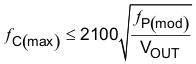For tantalum or aluminum capacitors use Equation 44:

Equation 44.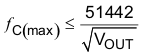For all cases use Equation 45 and Equation 46:

Equation 45.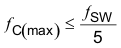Equation 46.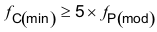When a crossover frequency, ƒC, has been selected, the gain of the modulator at the crossover frequency is calculated. The gain of the modulator at the crossover frequency is calculated using Equation 47.

Equation 47.For the example problem, the gain of the modulator at the crossover frequency is 0.542. Next, the compensation components are calculated. A resistor in series with a capacitor is used to create a compensating zero. A capacitor in parallel to these two components forms the compensating pole. However, calculating the values of these components varies depending on if the ESR zero is located above or below the crossover frequency. For ceramic or low ESR tantalum output capacitors, the zero will usually be located above the crossover frequency. For aluminum electrolytic and tantalum capacitors, the modulator zero is usually located lower in frequency than the crossover frequency. For cases where the modulator zero is higher than the crossover frequency (ceramic capacitors).

Equation 48.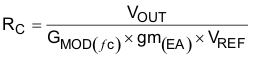Equation 49.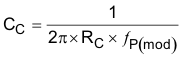Equation 50.For cases where the modulator zero is less than the crossover frequency (Aluminum or Tantalum capacitors), the equations are as follows:

Equation 51.Equation 52.Equation 53.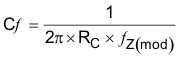For the example problem, the ESR zero is located at a higher frequency compared to the crossover frequency so Equation 50 through Equation 53 are used to calculate the compensation components. In this example, the calculated components values are:

• RC= 76.2 kΩ
• CC= 2710 pF
• Cƒ =6.17 pF

The calculated value of the Cƒ capacitor is not a standard value so a value of 2700 pF is used. 6.8 pF is used for CC. The RC resistor sets the gain of the error amplifier which determines the crossover frequency. The calculated RC resistor is not a standard value, so 76.8 kΩ is used.

#### 8.2.3 Application Curves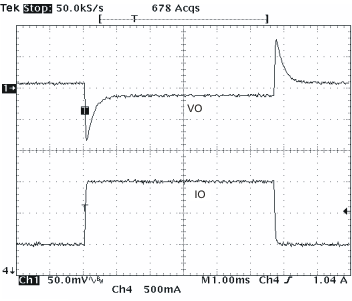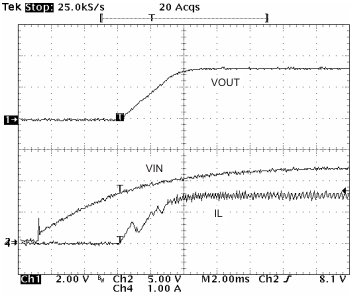Figure 54. VIN Power Up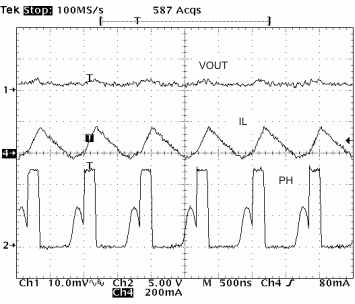Figure 56. Output Ripple, DCM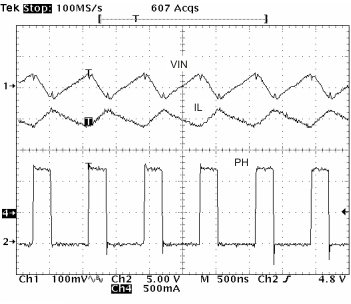Figure 58. Input Ripple CCM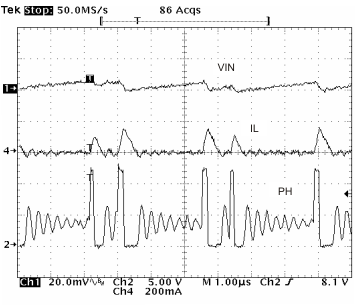Figure 60. Input Ripple PSM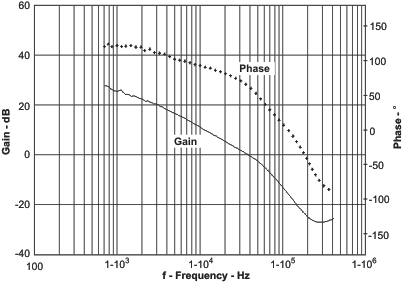Figure 62. Overall Loop Frequency Response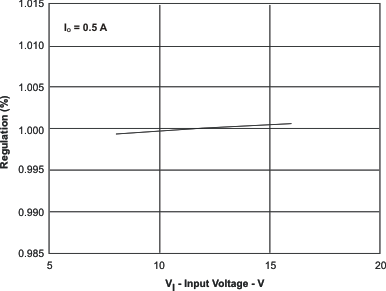Figure 64. Regulation vs Input Voltage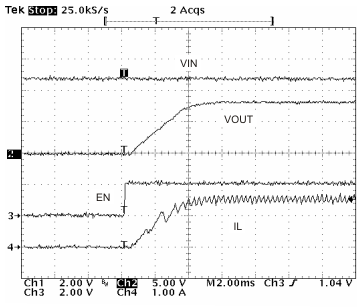Figure 53. Startup With ENFigure 55. Output Ripple CCM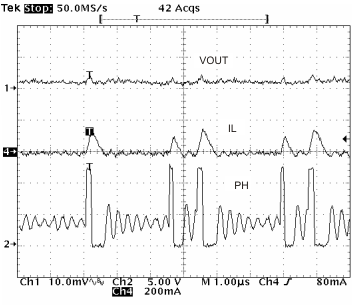Figure 57. Output Ripple, PSMFigure 59. Input Ripple DCMFigure 61. Efficiency vs Load Current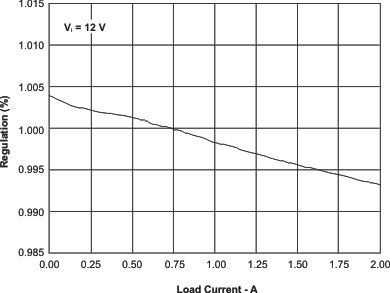Figure 63. Regulation vs Load Current